### William A. Link and Richard J. Barker. 2006. Model weights and the foundations of multimodel inference. Ecology 87:2626–2635.

Appendix A. Inconsistency of posterior mean when prior depends on sample size.

As an illustration of the consequences of having priors depend on sample size, consider the case of a Binomial random variable X consisting of N independent Bernoulli trials with success parameter p. Suppose p has a beta prior distribution with parameters a and b, denoted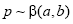. The posterior distribution of p is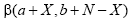with mean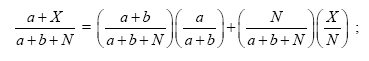this is a weighted average of the prior mean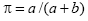and the maximum likelihood estimator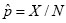The important feature is that as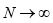, the weight on the prior mean goes to zero, provided that a and b are fixed.

Now suppose that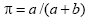is fixed, but that (a + b) = kN, for a fixed value of k. Then the posterior mean becomes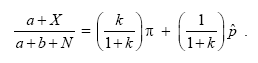The weight on the prior mean does not go to zero as. Indeed, the posterior mean converges to something different than the MLE, hence is not consistent.

[Back to E087-159]